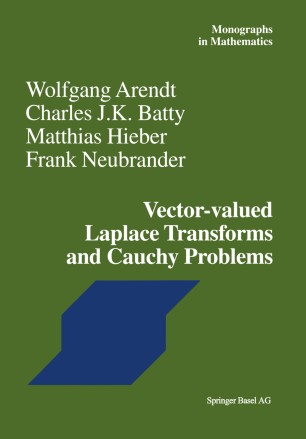# Vector-valued Laplace Transforms and Cauchy Problems

• Wolfgang Arendt
• Charles J. K. Batty
• Matthias Hieber
• Frank NeubranderBook

Part of the Monographs in Mathematics book series (MMA, volume 96)

1. Front Matter
Pages i-xi
2. ### Laplace Transforms and Well-Posedness of Cauchy Problems

1. Front Matter
Pages 1-4
2. Wolfgang Arendt, Charles J. K. Batty, Matthias Hieber, Frank Neubrander
Pages 5-63
3. Wolfgang Arendt, Charles J. K. Batty, Matthias Hieber, Frank Neubrander
Pages 65-108
4. Wolfgang Arendt, Charles J. K. Batty, Matthias Hieber, Frank Neubrander
Pages 109-240
3. ### Tauberian Theorems and Cauchy Problems

1. Front Matter
Pages 241-244
2. Wolfgang Arendt, Charles J. K. Batty, Matthias Hieber, Frank Neubrander
Pages 245-332
3. Wolfgang Arendt, Charles J. K. Batty, Matthias Hieber, Frank Neubrander
Pages 333-390
4. ### Applications and Examples

1. Front Matter
Pages 391-393
2. Wolfgang Arendt, Charles J. K. Batty, Matthias Hieber, Frank Neubrander
Pages 395-410
3. Wolfgang Arendt, Charles J. K. Batty, Matthias Hieber, Frank Neubrander
Pages 411-422
4. Wolfgang Arendt, Charles J. K. Batty, Matthias Hieber, Frank Neubrander
Pages 423-454
5. Back Matter
Pages 455-523

### Introduction

Linear evolution equations in Banach spaces have seen important developments in the last two decades. This is due to the many different applications in the theory of partial differential equations, probability theory, mathematical physics, and other areas, and also to the development of new techniques. One important technique is given by the Laplace transform. It played an important role in the early development of semigroup theory, as can be seen in the pioneering monograph by Rille and Phillips [HP57]. But many new results and concepts have come from Laplace transform techniques in the last 15 years. In contrast to the classical theory, one particular feature of this method is that functions with values in a Banach space have to be considered. The aim of this book is to present the theory of linear evolution equations in a systematic way by using the methods of vector-valued Laplace transforms. It is simple to describe the basic idea relating these two subjects. Let A be a closed linear operator on a Banach space X. The Cauchy problern defined by A is the initial value problern (t 2 0), (CP) {u'(t) = Au(t) u(O) = x, where x E X is a given initial value. If u is an exponentially bounded, continuous function, then we may consider the Laplace transform 00 u(>. ) = 1 e-). . tu(t) dt of u for large real>. .

### Keywords

Bochner integral Evolution equations Fourier transform Laplace and Fourier transforms differential equation functional analysis partial differential equation

#### Authors and affiliations

• Wolfgang Arendt
• 1
• Charles J. K. Batty
• 2
• Matthias Hieber
• 3
• Frank Neubrander
• 4
1. 1.Angewandte AnalysisUniversität UlmUlmGermany
2. 2.St. John’s CollegeOxfordUK